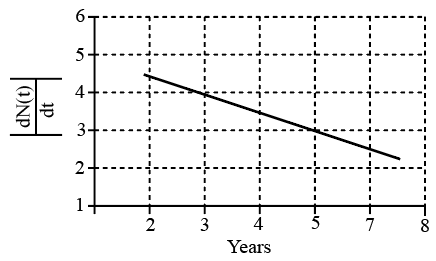Instructions

For the following questions answer them individually

Question 41

Question 42

Question 43

Question 44

Question 45

Backspace
789
456
123
0.-
Clear All
Question 46

Backspace
789
456
123
0.-
Clear All
Question 47

Backspace
789
456
123
0.-
Clear All
Question 48

Backspace
789
456
123
0.-
Clear All
Question 49

# To determine the half life of a radioactive element, a student plots a graph of $$\ln \mid \frac{dN(t)}{dt} \mid$$ versus t. Here $$\frac{dN(t)}{dt}$$ is the rate of radioactive decay at time t If the number of radioactive nuclei of this element decreases by a factor of p after 4.16 years, the value of p isBackspace
789
456
123
0.-
Clear All
Instructions

When liquid medicine of density $$\rho$$ is to be put in theeye, it is done with the help of a dropper. As the bulb onthe topof the dropperis pressed, a drop forms at the opening of the dropper. We wishto estimate the size of the drop. Wefirst assume that the drop formed at the opening is spherical because that requires a minimum increase in its surface energy. To determine the size, we calculate the net vertical force due to the surface tension T when the radius of the drop is R. When this force becomes smaller than the weight of the drop, the drop gets detached from the dropper.

Question 50

OR